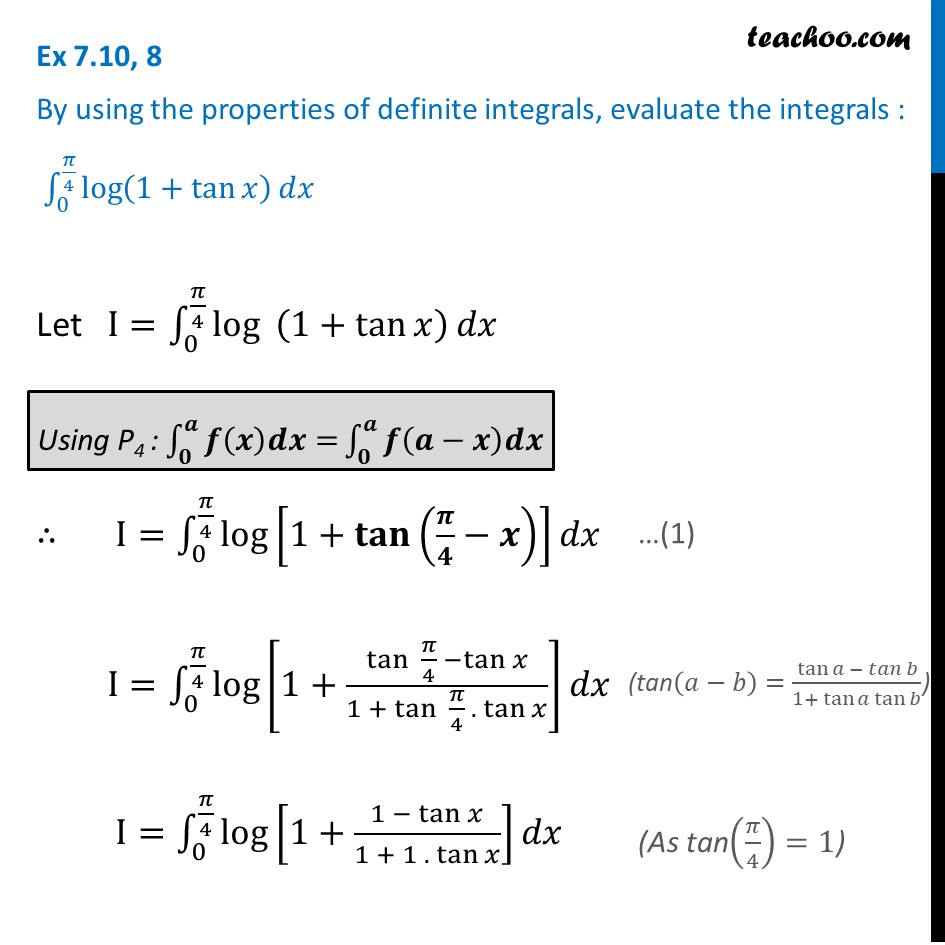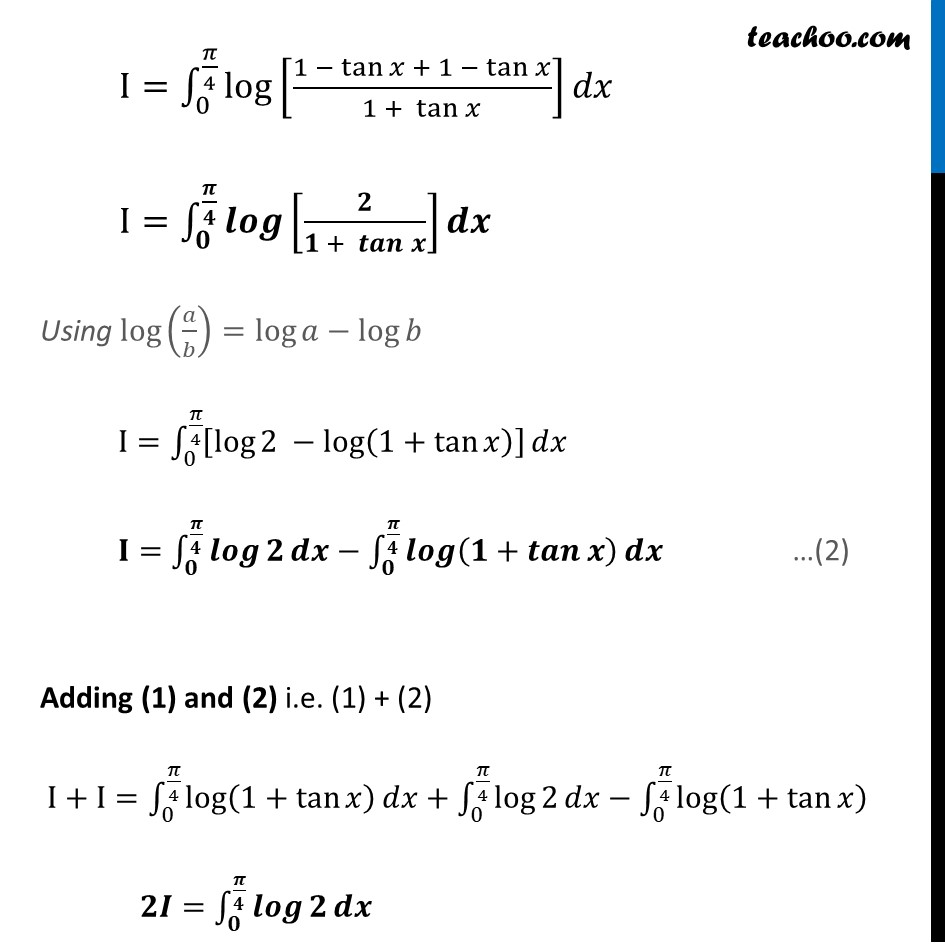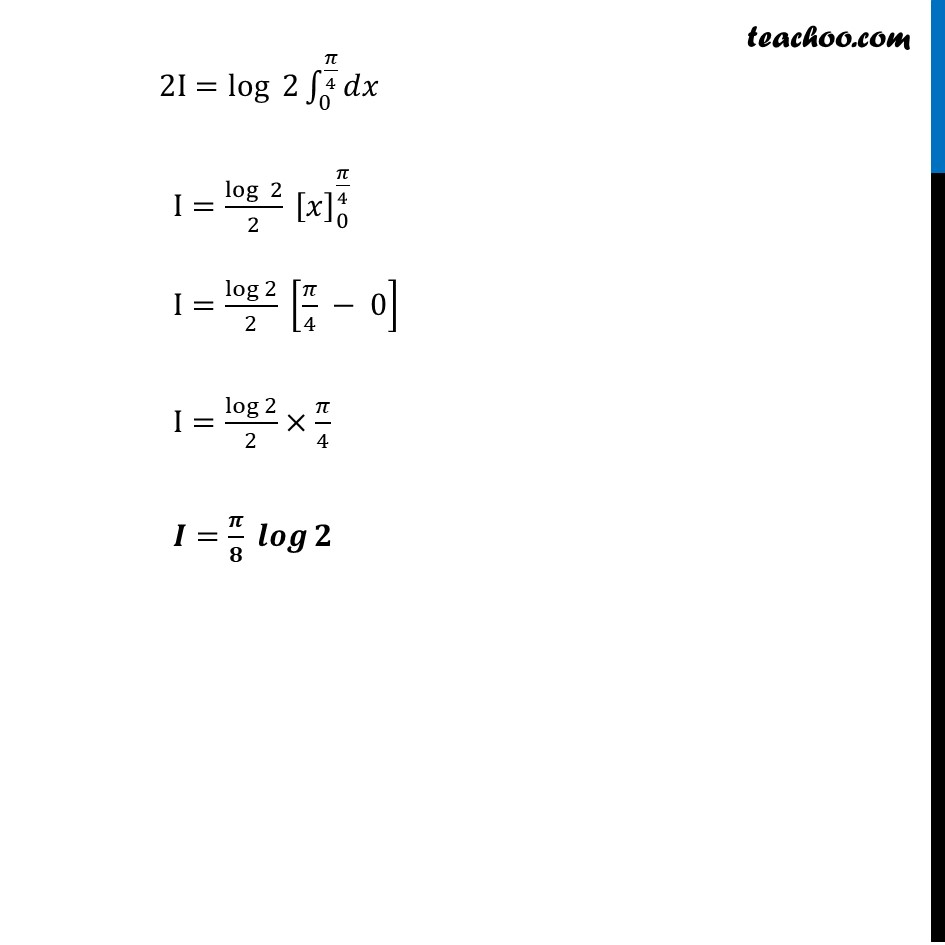Definite Integration by properties - P4

Chapter 7 Class 12 Integrals
Concept wiseLearn in your speed, with individual attention - Teachoo Maths 1-on-1 Class

### Transcript

Ex 7.10, 8 By using the properties of definite integrals, evaluate the integrals : ∫_0^(𝜋/4)▒log⁡(1+tan⁡𝑥 ) 𝑑𝑥 Let I=∫_0^(𝜋/4)▒log⁡〖 (1+tan⁡𝑥 )〗 𝑑𝑥 ∴ I=∫_0^(𝜋/4)▒log⁡[1+𝐭𝐚𝐧⁡(𝝅/𝟒−𝒙) ] 𝑑𝑥 I=∫_0^(𝜋/4)▒log⁡[1+(tan⁡ 𝜋/4 −tan⁡𝑥)/(1 +〖 tan〗⁡ 𝜋/4 . tan⁡𝑥 )] 𝑑𝑥 I=∫_0^(𝜋/4)▒log⁡[1+(1 − tan⁡𝑥)/(1 + 1 . tan⁡𝑥 )] 𝑑𝑥 I=∫_0^(𝜋/4)▒log⁡[(1 − tan⁡𝑥 + 1 − tan⁡𝑥)/(1 + tan⁡𝑥 )] 𝑑𝑥 I=∫_𝟎^(𝝅/𝟒)▒𝒍𝒐𝒈⁡[𝟐/(𝟏 + 𝒕𝒂𝒏⁡𝒙 )] 𝒅𝒙 Using log⁡(𝑎/𝑏)=log⁡𝑎−log⁡𝑏 I=∫_0^(𝜋/4)▒[log⁡2 −log⁡(1+tan⁡𝑥 ) ] 𝑑𝑥 𝐈=∫_𝟎^(𝝅/𝟒)▒𝒍𝒐𝒈⁡𝟐 𝒅𝒙−∫_𝟎^(𝝅/𝟒)▒𝒍𝒐𝒈⁡(𝟏+𝒕𝒂𝒏⁡𝒙 ) 𝒅𝒙 Adding (1) and (2) i.e. (1) + (2) I+I=∫_0^(𝜋/4)▒log⁡(1+tan⁡𝑥 ) 𝑑𝑥+∫_0^(𝜋/4)▒log⁡2 𝑑𝑥−∫_0^(𝜋/4)▒log⁡(1+tan⁡𝑥 ) 𝟐𝑰=∫_𝟎^(𝝅/𝟒)▒𝒍𝒐𝒈⁡𝟐 𝒅𝒙 2I=log⁡〖 2〗 ∫_0^(𝜋/4)▒𝑑𝑥 I=log⁡〖 2〗/2 [𝑥]_0^(𝜋/4) I=log⁡2/2 [𝜋/4 − 0] I=log⁡2/2×𝜋/4 𝑰=𝝅/𝟖 𝒍𝒐𝒈⁡𝟐